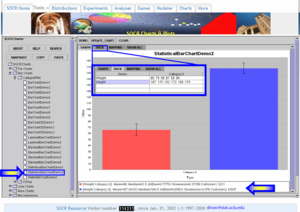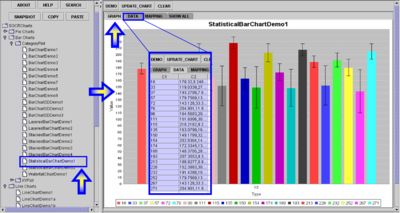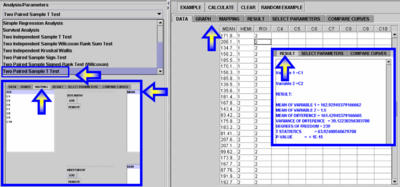# AP Statistics Curriculum 2007 IntroTools

General Advance-Placement (AP) Statistics Curriculum - Statistics with Tools

## Statistics with Tools (Calculators and Computers)

A critical component in any data analysis or process understanding approach is the development of a model. Models have compact analytical representations (e.g., formulas, symbolic equations, etc.) The model is frequently used to study the process theoretically. Empirical validation of the model is carried by plugging in data and actually testing the model. This validation step may be done manually, by computing the model prediction or model inference from recorded measurements. This typically may be done by hand only for small number of observations (<10). In practice, most of the time, we write (or use existent) algorithms and computer programs that automate these calculations for greater efficiency, accuracy and consistency in applying the model to larger datasets.

There are a number of statistical software tools (programs) that one can employ for data analysis and statistical processing. Some of these are: SAS, SYSTAT, SPSS, R, SOCR, etc.

## Approach & Model Validation

Before any statistical analysis tool is employed to analyze a dataset, one needs to carefully review the prerequisites and assumptions that this model demands about the data and study design.

For example, if we measure the weight and height of students and want to study gender, age or race differences or association between weight and height, we will need to make sure our sample size is large enough. These weight and height measurements are random (i.e., we do not have repeated measurements of the same student or twin-measurements) and that the students we measure are actually a representative sample of the population that we are making inference about (e.g., 8th-grade students). You can also find a real and large weight and height dataset here.

In this example, suppose we record the following 6 pairs of {weight (kg), height (cm)}:

 Student_Index 1 2 3 4 5 6 Weight 60 75 58 67 56 80 Height 167 175 152 172 166 175

We can easily compute the average weight (66 kg) and height (167 cm) using the sample mean-formula. We can also compute these averages using the SOCR Charts (BarChart3DDemo1), or any other statistical package, as shown in the image below.You can see a large dataset of human weights and heights here.

## Computational Resources: Internet-based SOCR Tools

Several of the SOCR tools and resources will be shown later, and will be useful in a various situations. Here is just a list of tools with one example of each:

## Hands-on Examples & Activities

### Data and Study Design

As part of a brain imaging study of Alzheimer's disease *, the investigators collected the following data. We will now demonstrate how computer programs, software tools and resources, like SOCR, can help in statistically analyzing larger datasets (certainly data size over 10 is difficult to calculate by hand correctly). In this case we'll work with 240 measurements derived from data acquired by this study.

### Data Plots

Let's first try to plot some of these data. Suppose we take a smaller fraction of the entire dataset. You can find a fragment of 21 rows and 3 columns of measurements here. This number is large enough to require computer software to graph the data. In column 1, this data subset includes an index of the region (blob) and in column 2, a pair of MEAN & Standard Deviation for the intensities over the blob (within the Left Occipital lobe). Now go to SOCR Charts and select the StatisticalBarChartDemo1 Chart (under BarCharts --> CategoryPlot), see figure below). Clear the default data and Paste in this data segment. Map the first column (C1) to Series and the second column (C2) to Categories and click UPDATE to redraw the graph with the new data. This plot shows the relations between the means and standard deviations of the intensities in the 21 regions (blobs, rows in table). We see that there is variation in both means and standard deviations (error bars on the box plots).### Statistical Analysis

Now we can demonstrate the use of SOCR Analyses to look for Left-Right hemispheric (HEMISPHERE) effects of the average MRI intensities (MEAN) in one Region of Interest (Occipital lobe, ROI=2). For this, we can apply simple Paired T-test. This analysis is justified as the average intensities that will follow Normal Distribution by the Central Limit Theorem because the left and right hemispheric observations are naturally paired.

• Copy in your mouse buffer the 6th (MEAN), 8th (HEMISPHERE) and 9th (ROI) columns of the following data table. You can paste these three columns in Excel, or any other spreadsheet program, and reorder the rows first by ROI and then by HEMISPHERE. This will give you an exert of 240 rows of measurements (MEAN) for ROI=2 (Occipital lobe) for each of the two hemispheres. The breakdown of this number of observations is as follows 240 = 2(hemispheres) * 3 (3D spatial locations, blobs) * 40 (Patients).
• Copy these 240 Rows and paste them in the Paired T-test Analysis under SOCR Analyses. Map the MEAN and HEMISPHERE columns to Dependent and Independent variables and then click Calculate. The results indicate that there are significant differences between the Left and Right Occipital mean intensities for these 40 subjects.